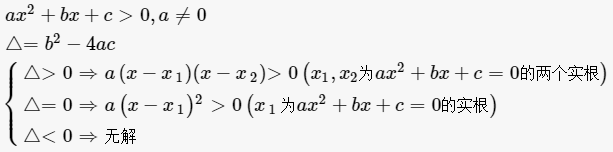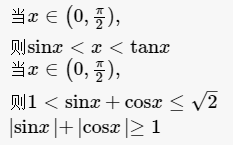y=sinx y'=cosx y=cosx y'=-sinx y=tanx y'=1/cos²x =sec²x y=cotx y'= -1/sin²x= - csc²x y=secx y'=secxtanx y=cscx y'=-cscxcotx y=arc.........